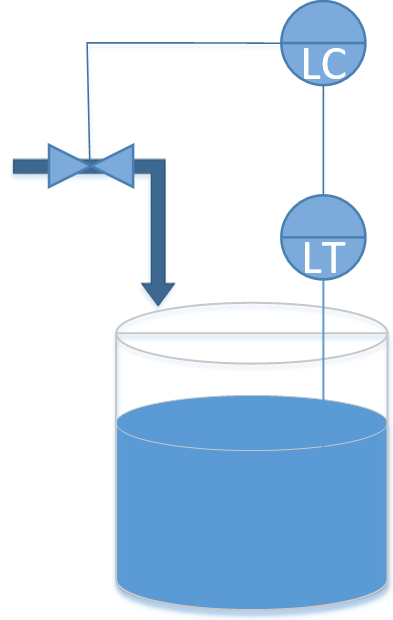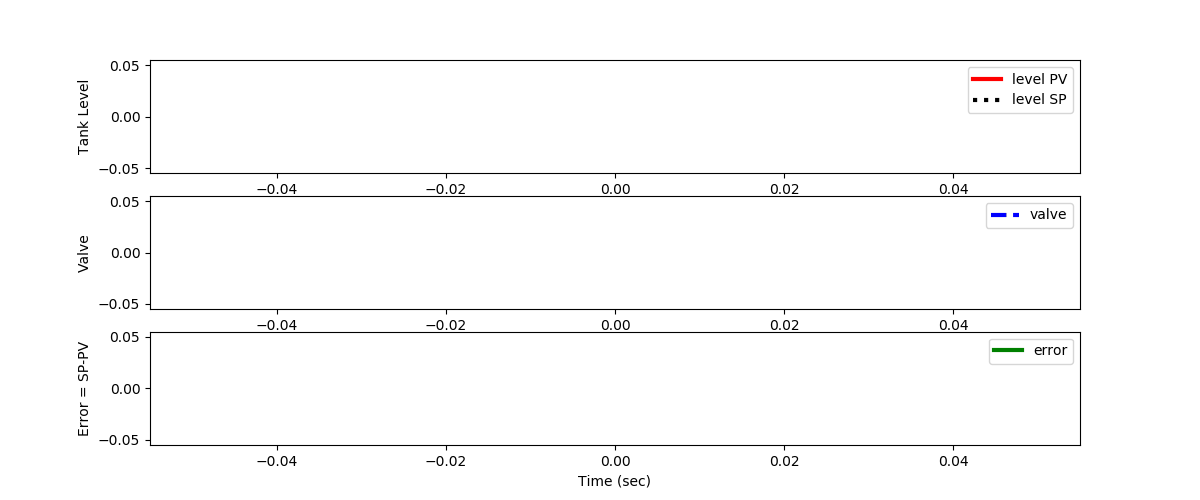Main

## Control a Water Tank Level

Consider a cylindrical tank with no outlet flow and an adjustable inlet flow that is controlled by a valve. The inlet flow rate is not measured but there is a level measurement that shows how much fluid has been added to the tank. The objective of this exercise is to develop a controller that can maintain a certain water level by automatically adjusting the inlet flow rate.Note: The symbol LT is an abbreviation for Level Transmitter. A concentration sensor is typically shown as CT for Concentration Transmitter or AT for Analyzer Transmitter. A temperature sensor such as a thermocouple is shown as TT which stands for Temperature Transmitter. If the second letter is C then it is a controller such as LC for Level Controller.

This example continues from the introduction to modeling where the process equation is derived and sample Python code is available.

$$\rho \; A \; \frac{dh}{dt} = c \; u \quad \mathrm{with} \quad \dot m_{in} = c \; u$$

where c is a constant that relates valve opening to inlet flow.

Design a P-only controller for the tank to maintain a level set point of 10.0 m. Test the P-only controller with different values of Kc by integrating the mass balance equation for a period of 10 seconds. Use a value of 1000 kg/m3 for density and 1.0 m2 for the cross-sectional area of the tank. For the valve, assume a valve coefficient of c=50.0 (kg/s / percent open). Make sure that the valve does not exceed the limits of 0-100 percent by clipping the requested valve opening to an acceptable range. For example, if the P-only controller calculates a valve opening of 150 percent, use 100 percent instead.

Use the following Python script as a starting point for the simulation and add the Proportional-only controller. Select "Get Code" from the link at the bottom corner to get unformatted text. Fill in the areas labeled "TO DO" to implement the P-only controller.

# it is recommended to run this script with IDLE that is available
# from Python.org
# animated plots need config changes in IPython (Anaconda)
# For Spyder (Anaconda) IDE, select the IDE menu bar:
#  select Tools, Preferences, IPython Console, Graphics, Backend=Automatic
# This will allow real-time plots to display as a new window instead of
#  inline in the IPython console.
import numpy as np
import matplotlib.pyplot as plt
from scipy.integrate import odeint

# animate plots?
animate=True # True / False

# define tank model
def tank(Level,time,c,valve):
rho = 1000.0 # water density (kg/m^3)
A = 1.0      # tank area (m^2)
# calculate derivative of the Level
dLevel_dt = (c/(rho*A)) * valve
return dLevel_dt

# time span for the simulation for 10 sec, every 0.1 sec
ts = np.linspace(0,10,101)

# valve operation
c = 50.0          # valve coefficient (kg/s / %open)
u = np.zeros(101) # u = valve % open

# level initial condition
Level0 = 0

# initial valve position
valve = 10

# for storing the results
z = np.zeros(101)
es = np.zeros(101)
sps = np.zeros(101)

# TO DO: what is the value for ubias?
# ubias = ?

# TO DO: decide on a tuning value for Kc
# Kc = ?

# TO DO: record the desired level (set point)
SP = 1

plt.figure(1,figsize=(12,5))
if animate:
plt.ion()
plt.show()

# simulate with ODEINT
for i in range(100):
# calculate the error
error = SP - Level0

# TO DO: put P-only controller here
# valve = 1

u[i+1] = valve   # store the valve position
es[i+1] = error  # store the error
y = odeint(tank,Level0,[0,0.1],args=(c,valve))
Level0 = y[-1] # take the last point
z[i+1] = Level0 # store the level for plotting
sps[i+1] = SP

if animate:
# update plot
plt.clf()
plt.subplot(3,1,1)
plt.plot(ts[0:i+1],z[0:i+1],'r-',linewidth=3,label='level PV')
plt.plot(ts[0:i+1],sps[0:i+1],'k:',linewidth=3,label='level SP')
plt.ylabel('Tank Level')
plt.legend(loc='best')
plt.subplot(3,1,2)
plt.plot(ts[0:i+1],u[0:i+1],'b--',linewidth=3,label='valve')
plt.ylabel('Valve')
plt.legend(loc='best')
plt.subplot(3,1,3)
plt.plot(ts[0:i+1],es[0:i+1],'g-',linewidth=3,label='error')
plt.ylabel('Error = SP-PV')
plt.xlabel('Time (sec)')
plt.legend(loc='best')
plt.pause(0.1)

if not animate:
# plot results
plt.subplot(3,1,1)
plt.plot(ts,z,'r-',linewidth=3,label='level PV')
plt.plot(ts,sps,'k:',linewidth=3,label='level SP')
plt.ylabel('Tank Level')
plt.legend(loc='best')
plt.subplot(3,1,2)
plt.plot(ts,u,'b--',linewidth=3,label='valve')
plt.ylabel('Valve')
plt.legend(loc='best')
plt.subplot(3,1,3)
plt.plot(ts,es,'g-',linewidth=3,label='error')
plt.ylabel('Error = SP-PV')
plt.xlabel('Time (sec)')
plt.legend(loc='best')
plt.show()

#### Solution# solution
import numpy as np
import matplotlib.pyplot as plt
from scipy.integrate import odeint

# animate plots?
animate=True # True / False

# define tank model
def tank(Level,time,c,valve):
rho = 1000.0 # water density (kg/m^3)
A = 1.0      # tank area (m^2)
# calculate derivative of the Level
dLevel_dt = (c/(rho*A)) * valve
return dLevel_dt

# time span for the simulation for 10 sec, every 0.1 sec
ts = np.linspace(0,10,101)

# valve operation
c = 50.0          # valve coefficient (kg/s / %open)
u = np.zeros(101) # u = valve % open

# level initial condition
Level0 = 0

# initial valve position
valve = 0

# for storing the results
z = np.zeros(101)
es = np.zeros(101)
sps = np.zeros(101)

# TO DO: what is the value for ubias?
ubias = 0

# TO DO: decide on a tuning value for Kc
Kc = 50.0

# TO DO: record the desired level (set point)
SP = 10

plt.figure(1,figsize=(12,5))
if animate:
plt.ion()
plt.show()
make_gif = True
try:
import imageio  # required to make gif animation
except:
print('install imageio with "pip install imageio" to make gif')
make_gif=False
if make_gif:
try:
import os
images = []
os.mkdir('./frames')
except:
print('Figure directory failed')
make_gif=False

# simulate with ODEINT
for i in range(100):
# calculate the error
error = SP - Level0

# TO DO: put P-only controller here
valve = ubias + Kc * error
valve = max(0,valve)
valve = min(100,valve)

u[i+1] = valve   # store the valve position
es[i+1] = error  # store the error
y = odeint(tank,Level0,[0,0.1],args=(c,valve))
Level0 = y[-1] # take the last point
z[i+1] = Level0 # store the level for plotting
sps[i+1] = SP

if animate:
# update plot
plt.clf()
plt.subplot(3,1,1)
plt.plot(ts[0:i+1],z[0:i+1],'r-',linewidth=3,label='level PV')
plt.plot(ts[0:i+1],sps[0:i+1],'k:',linewidth=3,label='level SP')
plt.ylabel('Tank Level')
plt.legend(loc='best')
plt.subplot(3,1,2)
plt.plot(ts[0:i+1],u[0:i+1],'b--',linewidth=3,label='valve')
plt.ylabel('Valve')
plt.legend(loc='best')
plt.subplot(3,1,3)
plt.plot(ts[0:i+1],es[0:i+1],'g-',linewidth=3,label='error')
plt.ylabel('Error = SP-PV')
plt.xlabel('Time (sec)')
plt.legend(loc='best')
filename='./frames/frame_'+str(1000+i)+'.png'
plt.savefig(filename)
if make_gif:
plt.pause(0.1)

if not animate:
# plot results
plt.subplot(3,1,1)
plt.plot(ts,z,'r-',linewidth=3,label='level PV')
plt.plot(ts,sps,'k:',linewidth=3,label='level SP')
plt.ylabel('Tank Level')
plt.legend(loc='best')
plt.subplot(3,1,2)
plt.plot(ts,u,'b--',linewidth=3,label='valve')
plt.ylabel('Valve')
plt.legend(loc='best')
plt.subplot(3,1,3)
plt.plot(ts,es,'g-',linewidth=3,label='error')
plt.ylabel('Error = SP-PV')
plt.xlabel('Time (sec)')
plt.legend(loc='best')
plt.show()
else:
# create animated GIF
if make_gif:
imageio.mimsave('animate.gif', images)